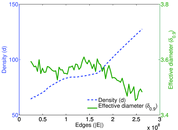# Delicious user–tag

This network contains user–tag relations from http://delicious.com/. Left nodes represent users, right nodes represent tags and an edge shows that a user tagged an URL using the tag represented by the right node.

 Code `Dut` Internal name `delicious-ut` Name Delicious user–tag Data source http://dai-labor.de/IRML/datasets AvailabilityDataset is available for download Consistency checkDataset passed all tests Category Interaction network Node meaning User, tag Edge meaning Tag assignment Network formatBipartite, undirected Edge typeUnweighted, multiple edges Temporal dataEdges are annotated with timestamps Snapshot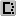Is a snapshot and likely to not contain all data

## Statistics

 Size n = 5,345,180 Left size n1 = 833,081 Right size n2 = 4,512,099 Volume m = 301,186,579 Unique edge count m̿ = 81,989,133 Wedge count s = 1,445,587,961,389 Claw count z = 58,243,254,727,040,968 Cross count x = 2.489 28 × 1021 Maximum degree dmax = 4,358,622 Maximum left degree d1max = 143,470 Maximum right degree d2max = 4,358,622 Average degree d = 112.695 Average left degree d1 = 361.533 Average right degree d2 = 66.750 9 Fill p = 2.181 17 × 10−5 Average edge multiplicity m̃ = 3.673 49 Size of LCC N = 5,339,821 Diameter δ = 14 50-Percentile effective diameter δ0.5 = 3.453 45 90-Percentile effective diameter δ0.9 = 4.588 55 Mean distance δm = 3.996 38 Gini coefficient G = 0.958 877 Balanced inequality ratio P = 0.064 980 4 Left balanced inequality ratio P1 = 0.191 035 Right balanced inequality ratio P2 = 0.045 447 5 Relative edge distribution entropy Her = 0.777 832 Power law exponent γ = 2.101 20 Tail power law exponent γt = 1.471 00 Degree assortativity ρ = −0.129 906 Degree assortativity p-value pρ = 0.000 00 Spectral norm α = 55,753.5

## Plots

### Degree distribution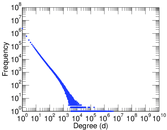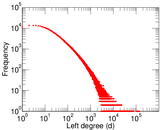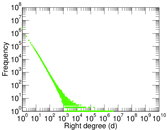### Cumulative degree distribution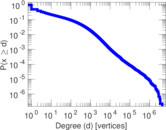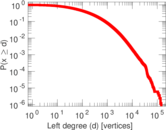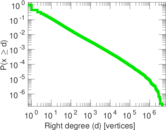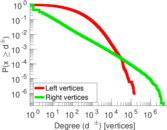### Lorenz curve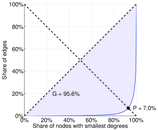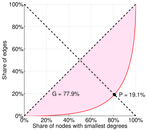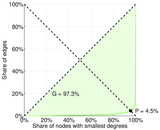### Spectral distribution of the adjacency matrix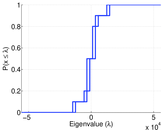### Spectral distribution of the normalized adjacency matrix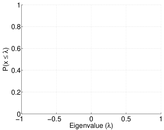### Spectral distribution of the Laplacian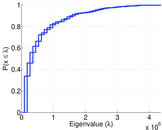### Spectral graph drawing based on the adjacency matrix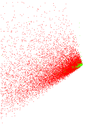### Spectral graph drawing based on the normalized adjacency matrix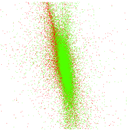### Degree assortativity### Hop distribution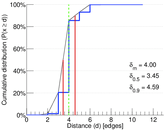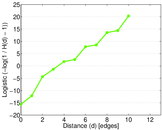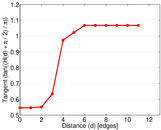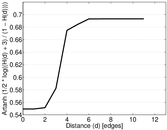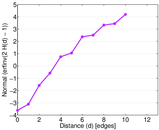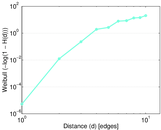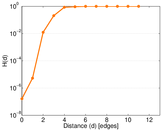### Edge weight/multiplicity distribution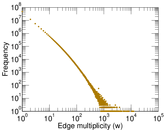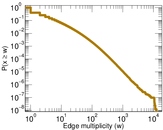### Temporal distribution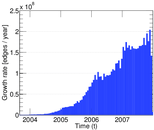### Diameter/density evolution